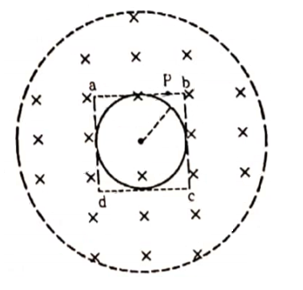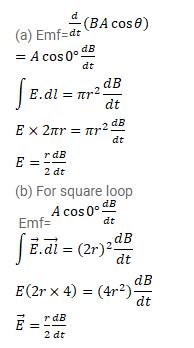# A uniform magnetic field B exists in a cylindrical region,

Question:

A uniform magnetic field B exists in a cylindrical region, shown dotted in figure. The magnetic field increases at a constant $\frac{d B}{d t}$

rate $^{d t}$. Consider a circle of radius $r$ coaxial with the cylindrical region.

(a) Find the magnitude of the electric field $E$ at a point on the circumference of the circle.

(b) Consider a point $\mathrm{P}$ on the side of the square circumscribing the circle. Show that the component of the induced electric field at $P$ along ba is the same as the magnitude found in part (a).Solution: